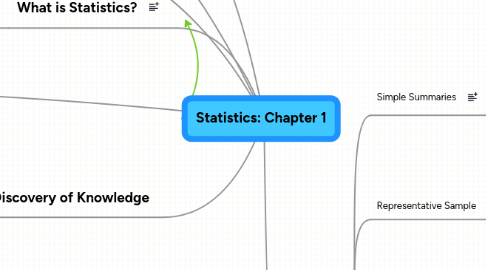Statistics: Chapter 1

Get Started. It's FreeStatistics: Chapter 16. Morals

6.1. Simple Summaries

6.1.1. Median

6.1.2. Lower Quartile

6.1.3. Upper Quartile

6.2. Representative Sample

6.2.1. Population

6.2.2. Random Sample

6.2.3. Sample Survey

6.2.4. Margin of Error

6.3. Unrepresentative Sample

6.3.1. Non Response Bias

6.3.2. Self-Selected (Volunteer) Sample

6.4. Observational Study

6.4.1. Variable

6.4.2. Confounding Variable

6.5. Randomized Experiments

6.5.1. Treatment

6.5.2. Random Assignment

6.5.3. Placebo

6.5.4. Statistical Significance

6.6. Rate and Risk

6.6.1. Base (Baseline) Risk